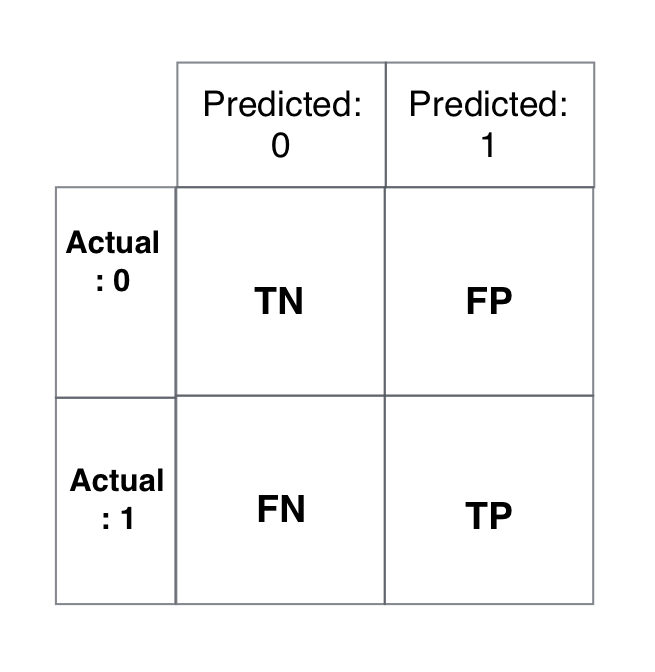Francisca Dias

## Introduction¶

In this paper I will focus on the evaluation metrics available for calssification problems using the dataset Costumer Churn.

Predicting when a costumer is about to churn is valuable in many businesses: communications, banking, movie rental, etc.

With the data at our disposal we can predict, using different models, who are the costumers that are more likely to churn.

My goal here is to show you different metrics and explain each one.

For this purposes I will leave out the results from other models, since I only want to focus in the meaning of results.

This is a binary classification problem, since the predicted results are Yes (1) or No (0).

Many classification models could be used here: KNeighbors Classifier, Logistic Regression, Gradient Boosting Classifier and so on.

I will only deploy the results from the Random Forest Classifier.

This dataset can be found here.

In :
import pandas as pd
import numpy as np

In :
churn_df = pd.read_csv('churn.csv')

In :
# Drop the columns that we have decided won't be used in prediction
churn_df = churn_df.drop(["Phone", "Area Code", "State"], axis=1)

In :
churn_df["Churn?"] = np.where(churn_df["Churn?"] == 'True.',1,0)

In :
churn_df["Int'l Plan"] = np.where(churn_df["Int'l Plan"] == 'yes',1,0)
churn_df["VMail Plan"] = np.where(churn_df["VMail Plan"] == 'yes',1,0)

In :
array = churn_df.values
X = array[:,0:17]

In :
from sklearn.preprocessing import StandardScaler

scaler = StandardScaler()
X = scaler.fit_transform(X)

In :
y = array[:,17]


## Classification Metrics¶

The metrics to evaluate the machine learning algorithms are very important.

The choice of metrics influence how you weight the importance of diferent characteristics in the results and your ultimate choice of which algorithm to choose.

Below are metrics that can be used to evaluate predictions on classification problems:

• Classification Accuracy
• Confusion Matrix
• Classification Report

## Classification Accuracy¶

• Classification accuracy tells us what is the number of correct predictions made as a ratio of all predictions made.
• Overall, how often is the classifier correct?
• Classification accuracy is the easiest classification metric to understand
• But, it does not tell you the underlying distribution of response values
• For this reason we should use other techniques such as the confusion matrix and classification report.
In :
from sklearn.model_selection import KFold
from sklearn.model_selection import cross_val_score

from sklearn.ensemble import RandomForestClassifier

model_rf = RandomForestClassifier()
kfold = KFold(n_splits=10, random_state=7)
results_rf = cross_val_score(model_rf, X, y, cv=kfold, scoring='accuracy')

In :
print('The results for Random Forest are', results_rf.mean())

The results for Random Forest are 0.949293305281


Here is the problem on using accuracy as a model performance measurement.

There are two scenarios that should be considered and addressed:

First it can happen that my classifier predicted a customer would churn and they didn't.

Second my classifier can predicted a customer would stay within the business, so nothing was done, and in the end they churned.

Second scenario is bad, and that is the one we will try to avoid by minimizing the value.

## Confusion Matrix¶

The confusion matrix is a handy presentation of the accuracy of a model with two or more classes.

We can draw a confusion matrix in two ways:

• We can call the function in sklearn by importing confusion_matrix or
• We can draw one ourselves, using just pandas: we use crosstab, and in the first argument we have the y test values and in the second argument we have the predicted y values.
In :
from IPython.display import Image
Image("conf_matrix.png")

Out:TN - True negatives : we predicted a costumer would not churn and they did not churn.

FP - False positives : We predicted a costumer would churn, but they did not.

FN - False negatives : we predicted a costumer would not churn and they actually churn.

TP - True positives : we predicted a costumer churn and they actually churn.

In :
from sklearn.model_selection import train_test_split

X_train, X_test, y_train, y_test = train_test_split(X, y, random_state=7)

model_rf.fit(X_train, y_train)
y_pred_rf = model_rf.predict(X_test)

In :
confusion_rf = pd.crosstab(y_test, y_pred_rf, rownames=['Actual'], colnames=['Predicted'], margins=True)
confusion_rf

Out:
Predicted 0.0 1.0 All
Actual
0.0 696 9 705
1.0 43 86 129
All 739 95 834

Here are some important questions that I can now answer:

• How often is the classifier correct? Accuracy (TP+TN)/total
• When an individual churns, how often does my classifier predict that correctly? Sensitivity or recall : (TP/actual yes)
• When a classifier predicts an individual will churn, how often does that individual actually churn? (TP/predicted yes )precision

Accuracy

In :
TP = 92
TN = 692
Total = 834
accuracy = (TP + TN)/Total
accuracy

Out:
0.9400479616306955

Sensitivity or Recall

In :
TP = 92
Actual_Yes = 129
sensitivity = TP / Actual_Yes
sensitivity

Out:
0.7131782945736435

Precision

In :
TP = 92
Predicted_Yes = 105
precision = TP / Predicted_Yes
precision

Out:
0.8761904761904762

## Classification Report¶

The scikit-learn library provides a great report when working on classification problems such as this one.

The Classification Report will give you a quick idea of the accuracy of a model using a number of measures:

• Precision
• Recall
• F1-score
In :
from sklearn.metrics import classification_report
print(classification_report(y_test, y_pred_rf))

             precision    recall  f1-score   support

0.0       0.94      0.99      0.96       705
1.0       0.91      0.67      0.77       129

avg / total       0.94      0.94      0.93       834